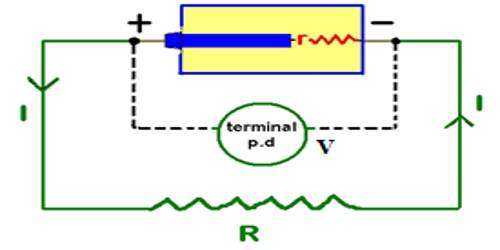# Electromotive Force of the Cell

Electromotive force of the cell

Due to the presence of positive and negative charges in the two terminals of the cell, a potential difference appears between the terminals. The maximum potential difference that exists between the terminals of the cell, when it is disconnected from the external circuit, is called the electromotive force of the cell. The electromotive force (EMF) is the maximum potential difference between two electrodes of a galvanic or voltaic cell. This quantity is related to the tendency for an element, a compound or an ion to acquire (i.e. gain) or release (loss) electrons.

If the cell is connected to the external resistance R1 [Fig], current will flow through R1. If the circuit is not disconnected or if the cell is not damaged current will continue to flow in the circuit. That means the cell is the driving force which maintains current flow in the circuit.So, the electromotive force may be defined in the following way.

The driving force which keeps or maintains the flow of current in the circuit is called electromotive force. It is denoted by ε or E.

Unit of emf: The unit of electromotive force is Joule/Coulomb (JC-1) or volt (V). But volt is extensively used as the unit. So, the unit of potential difference and electromotive force is the same.

Normally, emf of each of the cells used in a torch, E = 1.5V, i.e., chemical reaction inside the cell keeps the potential of the positive terminal 1.5V (or 15 JC-1) higher than, the potential of the negative terminal. In other words, a can be said that 1.5 J of work is done to bring back 1 coulomb of charge from a point of the circuit, through the cell to that point.